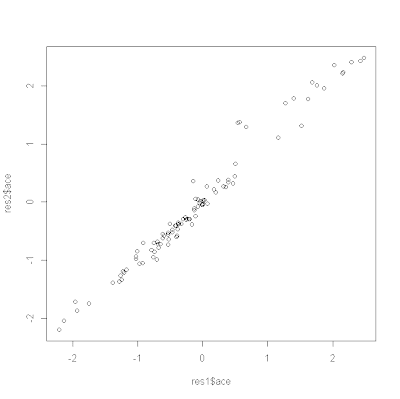## Tuesday, December 6, 2011

### Ancestral character estimation using likelihood

I just posted a new function for ancestral character estimation using likelihood called anc.ML(). (Direct link to code here.) This function is a lightweight version of ace() from the ape package, in that it only does likelihood estimation and only for continuously valued character traits. I developed this because I have been having trouble with ace(...,method="ML") in terms of accuracy. In particular, with some regularity (maybe 5% of the time on stochastic 100 taxon trees), the ML optimization in ace() seems to converge to the wrong ancestral character values.

One difficulty that I encountered when trying to evaluate the performance of anc.ML() was the discovery that the likelihoods reported by both functions are different. In particular, I realized that the likelihoods reported by ace() exclude constant terms. For a Brownian model, the constant terms for a set of ancestral states and rate for the BM process are two: –(n+m-1)log(2π)/2 (for n species and m internal nodes), and log(|C|)/2 (for cophenetic matrix C). Sure enough, this is exactly the amount by which the log-likelihoods returned by ace(...,method="ML") and anc.ML() differ.

First, an example when ace() is working:

> require(phytools) # needs phytools 0.1-2
> source("anc.ML.R") # load the source code for anc.ML
> set.seed(1)
> tree<-rtree(100)
> x<-fastBM(tree)
> res1<-ace(x,tree,method="ML")
> res1\$loglik
 -29.04148
> res2<-anc.ML(tree,x,maxit=4000)
> res2\$logLik
 -116.1555
> plot(res1\$ace,res2\$ace)
> res1\$loglik-(length(tree\$tip)+tree\$Nnode-1)*log(2*pi)/2-as.numeric(determinant(vcvPhylo(tree),logarithm=T)\$modulus)/2
 -116.1555This just shows that ace(...,method="ML") and anc.ML() produce the same estimates (to a fairly high degree of precision); and that the likelihoods differ by a constant for a given tree. That is, of course, when ace() behaves. Sometimes it doesn't. Try this example:

> set.seed(90)
> tree<-rtree(100)
> x<-fastBM(tree)
> res1<-ace(x,tree,method="ML")
> res1\$loglik-(length(tree\$tip)+tree\$Nnode-1)*log(2*pi)/2-as.numeric(determinant(vcvPhylo(tree),logarith=T)\$modulus)/2
 -92.44219
> res2<-anc.ML(tree,x,maxit=4000)
> res2\$logLik
 -84.40321
> plot(res1\$ace,res2\$ace)Obviously, something is amiss in this example. I was working on this to show how to do ancestral state estimation for a continuous trait when rates of evolution differ in different parts of the tree, and I will try to iron out any bugs in anc.ML as I work on this.

1.So, my friend Annat Haber and I were once having a discussion about how zero-length branches affect various analyses, as these are common byproducts of the various methods for timescaling paleontological trees. ace() complains that trees with zero-length branches have singular matrices, which really isn't true if you look at the eigenvalues of the vcv matrix (they are all positive).

For example:

set.seed(10)
tree<-rtree(10)
tree1<-tree;tree2<-tree
tree1\$edge.length<-0
tree2\$edge.length<-0
layout(matrix(1:3,,3));plot(tree);plot(tree1);plot(tree2) #I've collapsed a terminal edge in 1, an internal edge in 2
trait<-rnorm(10);names(trait)<-tree\$tip.label
x<-ace(trait,tree) #no error
x1<-ace(trait,tree1) #error!
x2<-ace(trait,tree2) #error!

I never got around to dissecting the code to find out why, however. So I tried your code.

y<-anc.ML(tree,trait)
y1<-anc.ML(tree1,trait)
y2<-anc.ML(tree2,trait)

Still complains the vcv matrix is singular. Maybe this is a problem with solve()?
-Dave

2.Hi Dave.

Singular matrices have a determinant of 0 and are non-invertible. In your example, vcv.phylo(tree1) is nonsingular, as you point out. However, ancestral character estimation uses the VCV matrix for all tips & internal nodes. (The phytools function that computes this is vcvPhylo.) In this case, the matrix will be singular if any terminal or internal edge lengths are zero. However, the matrix will not be singular in the latter case if we first collapse internal edges of zero length using multi2di(). (Unfortunately, anc.ML calls pic internally to get a starting estimate of σ so the present version will still fail, but for a different reason, if zero length internal edges are collapsed. I have fixed this and confirmed that anc.ML(multi2di(tree2),x) will work. I will post this updated version this afternoon.)